# The solar

The solar heating tank has the shape of a rotating cylinder. When the tank is in a horizontal position, the water in the tank wets 4/5 each tank bases. If we place the tank in a vertical position, the water in the tank will reach up to 1.2 m. Calculate the length of the tank.

x =  1.5 m

### Step-by-step explanation: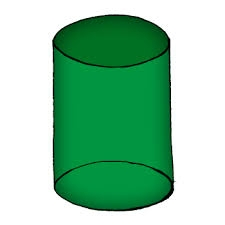Did you find an error or inaccuracy? Feel free to write us. Thank you!Tips to related online calculators
Need help to calculate sum, simplify or multiply fractions? Try our fraction calculator.
Tip: Our volume units converter will help you with the conversion of volume units.

## Related math problems and questions:

• The tankThe tank is full up to 4/5 of the total height and contains 240 hl of water. The area of the base is 6 square meters. What is the height of the tank?
• Cylindrical tankCylindrical tank holds 600hl water and is deep 2.5 m. Calculate the diameter of the cylinder.
• Stones in aquariumIn an aquarium with a length of 2 m, 1.5 m wide, and 2.5 m deep, the water is up to three-quarters of the depth. Can we place 2m cubic meters of stones in the aquarium without spilling water? (0 = no, 1 = yes)
• Water tank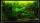The water tank shape cuboid has a width of 2.3 m and length twice larger. How high will reach water if water flows into 19 liters of water per second during 52 minutes?
• Cylindrical tank9.6 hl of water is poured into a cylindrical tank with a bottom diameter of 1.2 m. What height in centimeters does the water reach?
• Stones in aquariumIn an aquarium with a length 2 m; width 1.5 m and a depth of 2.5 m is a water level up to three-quarters of the depth. Can we place stones with a volume of 2 m3 into the aquarium without water being poured out?
• Water tankWater tank shape of cuboid has dimensions of the bottom 7.5 meters and 3 meters. How high will reach the water in the tank will flow 10 liters of water per second and the inflow will be open 5/6 hour? (Calculate to one decimal place and the results give i
• Tank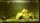The tank bottom has dimensions of 1.5 m and 3 2/6 m. The tank is 459.1 hl of water. How high is the water surface?
• The water tankThe water tank has the shape of a sphere with a radius of 2 m. How many liters of water will fit in the tank? How many kilograms of paint do we need to paint the tank, if we paint with 1 kg of paint 10 m2?
• TanksThe fire tank has a cuboid shape with a rectangular floor measuring 13.7 m × 9.8 m. Water depth is 2.4 m. Water was pumped from the tank into barrels with a capacity of 2.7 hl. How many barrels were used if the water level in the tank fallen 5 cm? W
• WaterInto a full cylindrical tank high 3 m with a base radius of 2.5 m, we insert cuboid with dimensions 1.7 m, 1.3 m, 1.9 m. How many liters of water will overflow out?
• The tankThe tank has 1320 liters of water. The tank has the shape of a prism, its base is an rectangle with sides a = 0,6 m and b = 1,5 m. How high does the water level reach in the tank?
• Top-open tank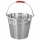The top-open tank has the shape of a truncated rotating cone, which stands on a smaller base. The tank's volume is 465 m3, the radii of the bases are 4 m and 3 m. Find the depth of the tank.
• Tom has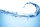Tom has a water tank that holds 5 gallons of water. Tom uses water from a full tank to fill 6 bottles that each hold 16 ounces and a pitcher that holds 1/2 gallon. How many ounces of water are left in the water tank?
• Cylinder containerThe cylindrical container with a diameter of 1.8 m contains 2,000 liters of water. How high does the water reach?
• The rainwaterThe rainwater container has the shape of a block whose bottom has dimensions of 4.5 m and 3.5 m. It is partly filled with water. What is the level if there is 189 hl of water in it?
• The well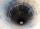The well has the shape of a cylinder with a diameter of 2 m. From ground level to water level are 4 meters and the depth of water in the well is 6 m. How many m3 of soil did they dig when digging a well? How many liters of water is in the well?